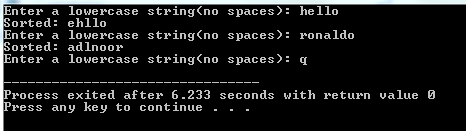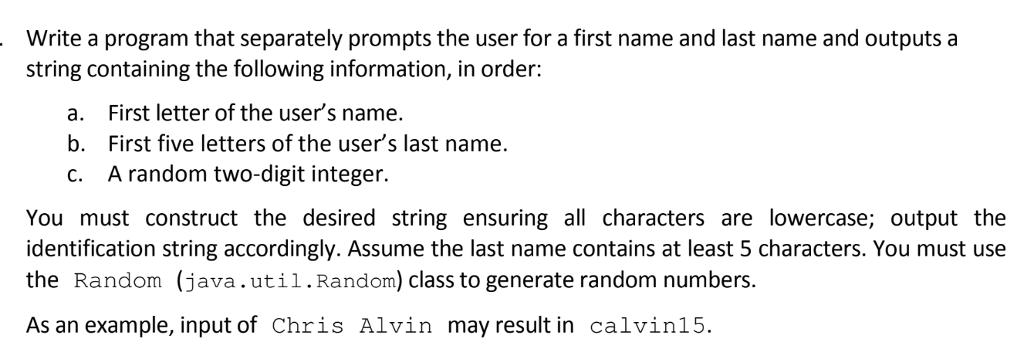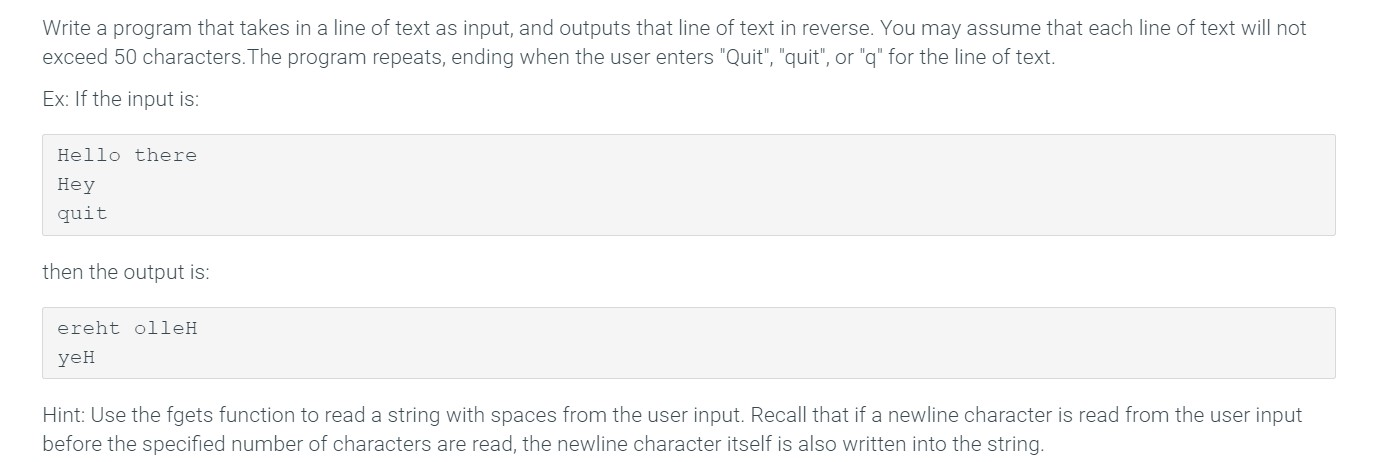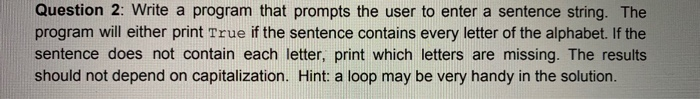Question

Write a C program that prompts the user for an input string with all lowercase letters. Your program should then sort the characters in the string and print the sorted string. Assume that the string contains no spaces and has at most 30 lowercase characters. Your program should loop continually until the user enters “q” to quit.

#include <stdio.h>
#include <string.h>

void sort(char arr[]) {
int len = strlen(arr);
int i, j;
char ch;
for(i = 0; i < len; ++i) {
for(j = 0; j < len-1; ++j) {
if(arr[j] > arr[j+1]) {
ch = arr[j];
arr[j] = arr[j+1];
arr[j+1] = ch;
}
}
}
}

int main()
{
char arr;
while(1) {
printf("Enter a lowercase string(no spaces): ");
scanf("%s", arr);
if(strcmp(arr, "q") == 0) {
break;
}
sort(arr);
printf("Sorted: %s\n", arr);
}
return 0;
}#### Earn Coins

Coins can be redeemed for fabulous gifts.

Similar Homework Help Questions
• ### Write a C program to “de-vowel” an input string. Assume that the user provides input containing...

Write a C program to “de-vowel” an input string. Assume that the user provides input containing only the characters a through z (i.e., all lowercase letters). Your program should create an output string that deletes all vowels from the input string, pushing the letters together to ﬁll any gaps. For example, given the input “theturtleandthehare” your code should print out “thtrtlndthhr”. Your program should create an output string from the input string, before printing its output. Sample Input: Enter a...

• ### Write a program that separately prompts the user for a first name and last name and...Write a program that separately prompts the user for a first name and last name and outputs a string containing the following information, in order: a. First letter of the user's name. b. First five letters of the user's last name. c. A random two-digit integer You must construct the desired string ensuring all characters are lowercase; output the identification string accordingly. Assume the last name contains at least 5 characters. You must use the Random (java.util.Random) class to generate...

• ### 1. Write a program that prompts the user to enter three integers and display the integers...

1. Write a program that prompts the user to enter three integers and display the integers in non-decreasing order. You can assume that all numbers are valid. For example: Input Result 140 -5 10 Enter a number: Enter a number: Enter a number: -5, 10, 140 import java.util.Scanner; public class Lab01 { public static void main(String[] args) { Scanner input = new Scanner(System.in);    } } ---------------------------------------------------------------------------------------------------------------------------- 2. Write a program that repeatedly prompts the user for integer values from...

• ### Write a program that takes in a line of text as input, and outputs that line...Write a program that takes in a line of text as input, and outputs that line of text in reverse. You may assume that each line of text will not exceed 50 characters. The program repeats, ending when the user enters "Quit", "quit", or "q" for the line of text. Ex: If the input is: Hello there Hey quit then the output is: ereht olleH уен Hint: Use the fgets function to read a string with spaces from the user...

• ### LANGUAGE = C i. Write a program that takes int numbers from user until user gives...

LANGUAGE = C i. Write a program that takes int numbers from user until user gives a sentinel value (loop terminating condition). Sort the numbers in ascending order using Insertion sort method. Sorting part should be done in different function named insertionSort(). Your code should count the number of swaps required to sort the numbers. Print the sorted list and total number of swaps that was required to sort those numbers. (4 marks) ii. In this part take another number...

• ### Write a Java program that will create an array of Strings with 25 elements. Input Strings...

Write a Java program that will create an array of Strings with 25 elements. Input Strings from the user and store them in the array until either the user enters “quit” or the array is full. HINT: the sentinel value is “quit” all lowercase. “Quit” or “QUIT” should not stop the loop. HINT: you have to use the equals method (not ==) when you are comparing two Strings. After the input is complete, print the Strings that the user entered...

• ### Answer in python please and use loop Question 2: Write a program that prompts the user...Answer in python please and use loop Question 2: Write a program that prompts the user to enter a sentence string. The program will either print True if the sentence contains every letter of the alphabet. If the sentence does not contain each letter, print which letters are missing. The results should not depend on capitalization. Hint: a loop may be very handy in the solution.

• ### s1 = 'Practice How to Use String Object' Write a python statement that splits the string,...

s1 = 'Practice How to Use String Object' Write a python statement that splits the string, creating the following list: ['Practice', 'How', 'to', 'Use', 'String', 'Object'] 2. Assume reply references a string. The following if statement determines whether reply is equal to ‘Y’ or ‘y’: reply = input("Y or y to continue: ") if reply == "Y" and reply == "y":    print("Y --- <uppercase> has been selected. ") else:    print("y --- <lowercase> has been chosen. ") print("… continue...

• ### Write a c++ program to accept user input of a long string with spaces that ends...

Write a c++ program to accept user input of a long string with spaces that ends with ‘\n’, and then calculate the sum of all even numbers in the string. You may assume the input string is less than 1000 characters, and all numbers are integers.

• ### In Java, write a program that prompts the user to input a sequence of characters and...

In Java, write a program that prompts the user to input a sequence of characters and outputs the number of vowels. You will need to write a method to return a value called isAVowel which will return true if a given character is a vowel and returns false if the character is not a vowel. A second method, called isAConstant, should return true if a given character is a constant and return false if the character is not a constant....